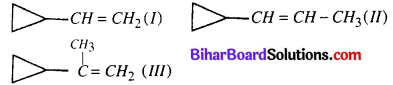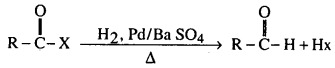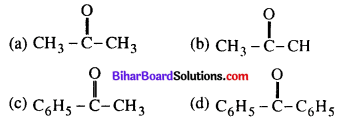Bihar Board 12th Chemistry Objective Questions and Answers

## Bihar Board 12th Chemistry VVI Objective Questions Model Set 1 in English

Question 1.
A dilute aqueous solution of sodium fluoride is electrolysed; the products at the anode and cathode are
(a) F2, Na
(b) F2, H2
(c) O2, Na
(d) O2, H2
(b) F2, H2

Question 2.
Number of σ and π bonds in C2 molecule is/are
(a) 1σ and 1π
(b) 1σ and 2π
(c) 2π only
(d) 1σ and 3π
(b) 1σ and 2πQuestion 3.
Rate of hydration in aqueous acid will be in the order(a) I < II < III
(b) III < II < I
(c) I < III < II
(d) II < I < III
(d) II < I < III

Question 4.
The molecule which has zero dipole moment is
(a) NF3
(b) BF3
(c) ClO2
(d) CH2Cl2
(b) BF3

Question 5.
The correct order of equivalent conductance at infinite dilution of LiCl, NaCl and KCl is
(a) LiCl > NaCl > KCl
(b) KCl > NaCl > LiCl
(c) NaCl > KCl > LiCl
(d) LiCl > KCl > NaCl
(b) KCl > NaCl > LiClQuestion 6.
R-OH + CH2N2 → Leaving group in this reaction is
(a) CH3
(b) R
(C) N2
(d) CH2
(a) CH3

Question 7.
The product obtained when silica reacts with hydrogen fluoride is
(a) SiF4
(b) H2SiF6
(c) H2SiF4
(d) H2SiF3
(b) H2SiF6

Question 8.
The van’t Hoffs factor of 0.1 M Ba(NO2)2 solution is 2.74. The degree of dissociation is
(a) 91.3%
(b) 87%
(c) 100%
(d) 74%
(b) 87%

Question 9.
Which of the following has the most stable +2 oxidation state?
(a) Sn
(b) Ag
(c) Fe
(d) Pb
(d) PbQuestion 10.
The compound having tetrahedral geometry is
(a) [NiCl4]2-
(b) [Ni(CN)4]2-
(c) [PdCl4]2-
(d) [NiCl4]2- and [PdCl4]2-
(a) [NiCl4]2-

Question 11.
The Cannizzaro’s reaction is not given by
(a) Formaldehyde
(b) Acetaldehyde
(c) Benzaldehyde
(d) Furfural
(b) Acetaldehyde

Question 12.
Which represents a zero-order reaction?Question 13.
Which gas is absorbed strongly by charcoal?
(a) CO
(b) NH3
(C) NCl3
(d) H2
(a) CO

Question 14.
If $$\frac{d x}{d t}$$ = K[H+]n and rate becomes 100 times when pH changes from 2 to 1, the order of reaction is
(a) 1
(b) 2
(c) 3
(d) 0
(c) 3Question 15.
Equivalent conductance of saturated BaSO4 is 400 ohm-1 cm2 eqvt-1 and specific conductance is 8 × 10-5 ohm-1. The Ksp of BaSO4 is
(a) 4 × 10-8 M2
(b) 10-8 M2
(c) 2 × 10-4 M2
(d) 10-4 M2
(c) 2 × 10-4 M2

Question 16.
An organic compound gives the iodoform test and also gives a positive test with Tollens reagent. The compound is(c) CH3 – CH2OH

Question 17.
IUPAC name of H2[Pt Cl6] is
(a) Hydrogen hexachloro platinate (IV)
(b) Hydrogen hexachloro platinate (II)
(c) Hydgoren Hexa chloride Pt (IV)
(d) Hydrogen Hexa chloride Pt (II)
(d) Hydrogen Hexa chloride Pt (II)

Question 18.
Mercuric chloride reacts with ammonia gas and forms a white precipitate. The molecular formula of the white precipitate is
(a) HgCl2. 2NH3
(b) Hg(NH3)2 Cl2
(c) Hg(NH2) Cl2
(d) Hg(NH2) Cl
(a) HgCl2. 2NH3

Question 19.
Which alkyl halide follows only SN2 hydrolysis mechanism?
(a) CH3 – CH2 – X(c) (CH3)3 C – X
(d) C6H5 – CH2X
(c) (CH3)3 C – XQuestion 20.Half-life of this reaction is independent of concentration of reactant. After 10 minutes volume of N2 gas is 10 litre and after complete reaction 100 litre. The rate constant of the reaction in min-1 unit is
(a) $$\frac{2.303}{10}$$
(b) $$\frac{2.303}{10}$$ log 5.0
(c) $$\frac{2.303}{10}$$ log 2.0
(d) $$\frac{2.303}{10}$$ log 4.0
(b) $$\frac{2.303}{10}$$ log 5.0

Question 21.
When NaCl solution is added to Fe(OH)3 colloidal solution then
(a) [Fe(OH3)] Fe3+ is formed
(b) [Fe(OH3)] Cl is formed
(c) [Fe(OH3)] Na+ is formed
(d) Fe(OH)3 is formed
(a) [Fe(OH3)] Fe3+ is formed

Question 22.
Which complex has a maximum paramagnetic moment value amongst the following
(a) [Cr(H2O)6]3+
(b) [Fe(H2O)6]Cl2
(c) [Fe(CN)6]4-
(d) [Ni(CO)4]
(d) [Ni(CO)4]

Question 23.
Which of the following gives a yellow or brown precipitate with alkaline Nessler’s reagent?
(a) CO2
(b) NH3
(c) NaCl
(d) KI
(b) NH3Question 24.
A 5% solution of cane sugar (Mol. Wt = 342) is isotonic with 1 % solution of substance X. The molecular weight of X is
(a) 68.4
(b) 34.2
(c) 171.2
(d) 136.2
(b) 34.2

Question 25.(a) 1.36 V
(b) 1.30 V
(c) 1.42 V
(d) 1.20 V
(c) 1.42 V

Question 26.
An Organic compound reacts with benzene sulfonyl chloride and product dissolves in aqueous NaOH. The compound is
(a) R – NH2(d) all of these
(a) R – NH2

Question 27.
A compound on hydrolysis gives 1°- amine. The compound is
(a) anilide
(b) amide
(c) cyanide
(d) None
(c) cyanideQuestion 28.This reaction is known as
(a) Stephen’s reaction
(b) Cannizzaro’s reaction
(c) Rosenmund’s reaction
(d) Hinsberg’s reaction
(c) Rosenmund’s reaction

Question 29.
Percentage of free space in bcc. unit cell is
(a) 32%
(b) 34%
(c) 28%
(d) 30%
(a) 32%

Question 30.
The coordination number of a metal crystallizing in hexagonal close-packed (hcp) structure is
(a) 12
(b) 8
(c) 4
(d) 6
(a) 12Question 31.
Which of the following compound is most reactive towards nucleophilic addition?Question 32.
Copper sulphate solution on treatment with an excess of KI gives whitish precipitate. The precipitate
(a) CuI2
(b) Cu2I2
(c) Cu2SO4
(d) I2
(b) Cu2I2

Question 33.
The most stable oxidation state of bismuth is
(a) +3
(b) +5
(c) +3 and +5 both
(d) None
(a) +3

Question 34.
Which metal nitrate gives colourless gas on thermal decomposition?
(a) NaNO3
(b) Cu(NO3)2
(c) Ba(NO3)2
(d) Hg(NO3)2